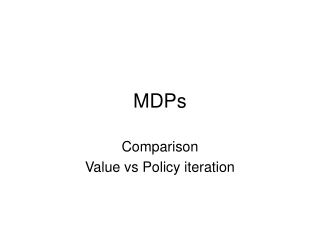DownloadDownload PresentationMDPs

# MDPs

Download Presentation## MDPs

- - - - - - - - - - - - - - - - - - - - - - - - - - - E N D - - - - - - - - - - - - - - - - - - - - - - - - - - -
##### Presentation Transcript

1. MDPs Comparison Value vs Policy iteration

2. Value iteration • two important properties of contractions: (i)A contraction has only one fixed point; if there were two fixed points they would not get closer together when the function was applied, so it would not be a contraction. (ii)When the function is applied to any argument, the value must get closer to the fixed point (because the fixed point does not move), so repeated application of a contraction always reaches the fixed point in the limit. 2

3. Value iteration • Let Ui denote the vector of utilities for all the states at the ith iteration. Then the Bellman update equation can be written as 3

4. Value iteration use the max norm, which measures the length of a vector by the length of its biggest component: Let Ui and Ui' be any two utility vectors. Then we have 4

5. Value iteration • In particular, we can replace U,' in Equation (17.7) with the true utilities U, for which B U = U. Then we obtain the inequality • Next Figure shows how N varies with y, for different values of the ratio 5

6. Value iteration From the contraction property (Equation (17.7)), it can be shown that if the update is small (i.e., no state's utility changes by much), then the error, compared with the true utility function, also is small. More precisely, 7

7. Value iteration • policy loss Uπi (s) is the utility obtained if πi is executed starting in s, policy lossis the most the agent can lose by executing πi instead of the optimal policy π* 8

8. Value iteration The policy loss of πi is connected to the error in Ui by the following inequality: 9

9. Policy iteration • The policy iteration algorithm alternates the following two steps, beginning from some initial policy π0 : • Policy evaluation: given a policy πi, calculate Ui = Uπi, the utility of each state if πi were to be executed. • Policy improvement: Calculate a new MEU policy πi+1, using one-step look-ahead based on Ui (as in Equation (17.4)). 10

10. Policy iteration • For n states, we have n linear equations with n unknowns, which can be solved exactly in time O(n3) by standard linear algebra methods. For large state spaces, O(n3) time might be prohibitive • modified policy iteration The simplified Bellman update for this process 12

11. Policy iteration In fact, on each iteration, we can pick any subset of states and apply either kind of updating (policy improvement or simplified value iteration) to that subset. This very general algorithm is called asynchronous policy iteration. 13